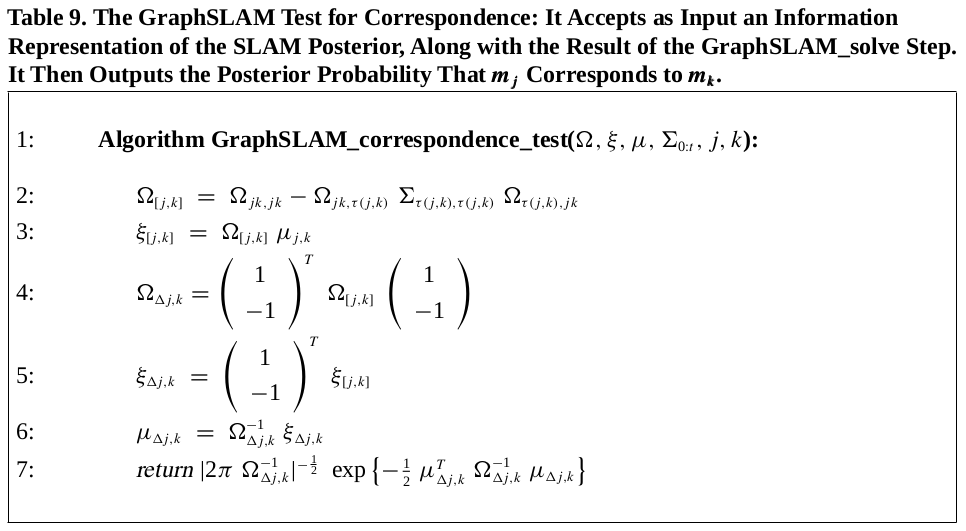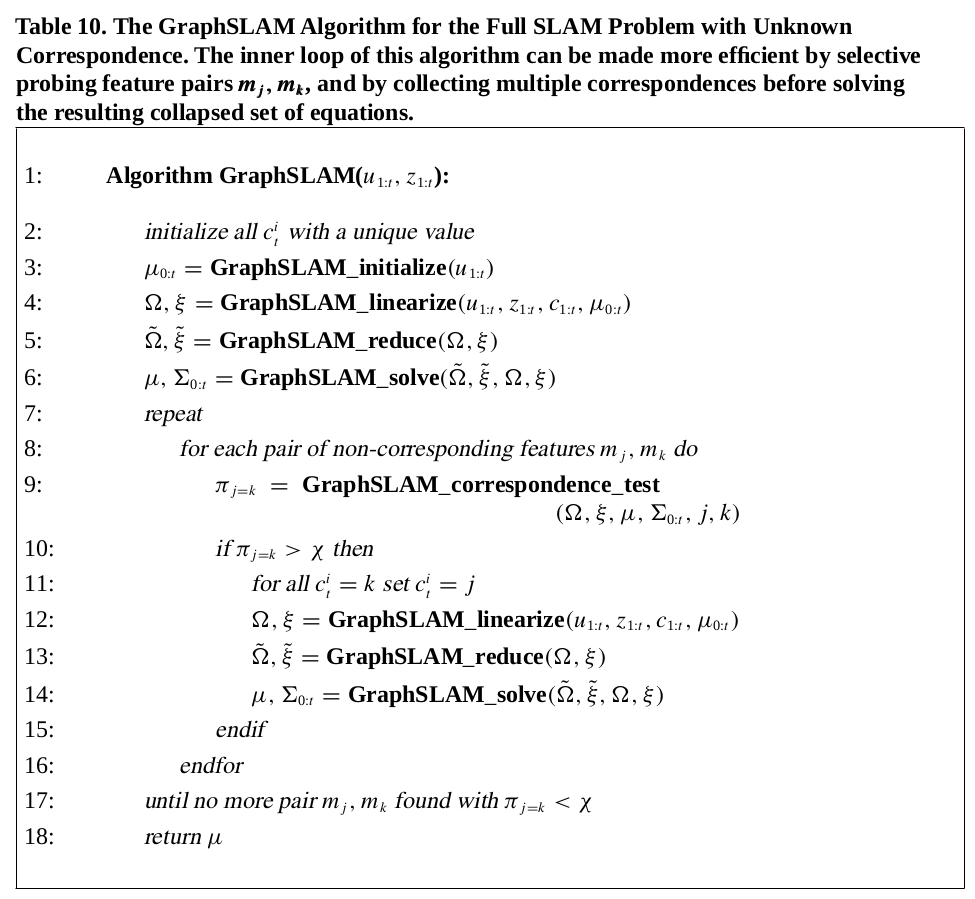0%

### GraphSLAM 中的数据处理

• 如果 $m_j$ 和 $m_k$ 是世界中的同一个物理点，则有 $c(j,k) = 1$
• 否则，$c(j,k) = 0$

• 在搜索过程中， GraphSLAM 可以考虑任何特征点的集合，而不用考虑他们出现的顺序。
• 匹配搜索可以和求解地图同时进行。假设两个两个搜索到的特征点关联了同一个实体点，通过将这个匹配假设引入地图中，会对地图其他匹配假设有影响。
• GraphSLAM 中的数据处理决定可以不做完。数据处理的优劣取决其他对其他数据的处理；对于某一个最初是较优的选择的匹配在后续可能会发现其实不好，而 GraphSLAM 可以随时修改（去除）这个匹配关系。

#### 对未知匹配关系的 GraphSLAM 算法

• 信息矩阵和信息向量表示的 SLAM 的后验概率
• Graph_solve 的求解结果 位姿和特征点的均值$\mu$ 和位姿的方差 $\Sigma_{0:t}$• 计算两个特征点之间的边际后验概率。用 $\Omega{[j,k]}$ 和 $\xi{[j,k]}$ 来表示。这一步的原理也是根据上一篇笔记中提到的边缘化引理，详见：The GraphSLAM algorithm with applications to large scale mapping of urban structures: Part 3
• 接下来，算法计算新的高斯随机变量 $\Delta{j,k} = m_j - m_k$的参数，即信息参数 $\Omega{\Delta{j,k}}, \xi{\Delta_{j,k}}$
• 最后计算差值变量的期望，最后一行返回 $m_j$ 和 $m_k$ 相差为 0 的概率。• 首先对匹配关系进行了初始化（每个观测值赋予一个独特值）
• 在重复迭代求解的过程，加入了一步对匹配关系的测试，对于特征点集合中任意两个不匹配（尚未匹配）的点进行匹配性测试如果匹配性高于一个阈值则设为匹配，重复进行求解，直到没有出现新的匹配关系为止

• 它测试了所有的特征点的点，而没有考虑距离等因素;
• 并且每当找到一个新的匹配关系就重新地图重建，而不是对多个新的匹配关系进行统一重建